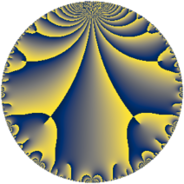Properties

 Label 9.6.aLevel $9$ Weight $6$ Character orbit 9.a Rep. character $\chi_{9}(1,\cdot)$ Character field $\Q$ Dimension $1$ Newform subspaces $1$ Sturm bound $6$ Trace bound $0$

Related objects

Defining parameters

 Level: $$N$$ $$=$$ $$9 = 3^{2}$$ Weight: $$k$$ $$=$$ $$6$$ Character orbit: $$[\chi]$$ $$=$$ 9.a (trivial) Character field: $$\Q$$ Newform subspaces: $$1$$ Sturm bound: $$6$$ Trace bound: $$0$$

Dimensions

The following table gives the dimensions of various subspaces of $$M_{6}(\Gamma_0(9))$$.

Total New Old
Modular forms 7 2 5
Cusp forms 3 1 2
Eisenstein series 4 1 3

The following table gives the dimensions of the cuspidal new subspaces with specified eigenvalues for the Atkin-Lehner operators and the Fricke involution.

$$3$$Dim.
$$-$$$$1$$

Trace form

 $$q + 6 q^{2} + 4 q^{4} - 6 q^{5} - 40 q^{7} - 168 q^{8} + O(q^{10})$$ $$q + 6 q^{2} + 4 q^{4} - 6 q^{5} - 40 q^{7} - 168 q^{8} - 36 q^{10} + 564 q^{11} + 638 q^{13} - 240 q^{14} - 1136 q^{16} - 882 q^{17} - 556 q^{19} - 24 q^{20} + 3384 q^{22} + 840 q^{23} - 3089 q^{25} + 3828 q^{26} - 160 q^{28} - 4638 q^{29} + 4400 q^{31} - 1440 q^{32} - 5292 q^{34} + 240 q^{35} - 2410 q^{37} - 3336 q^{38} + 1008 q^{40} + 6870 q^{41} + 9644 q^{43} + 2256 q^{44} + 5040 q^{46} + 18672 q^{47} - 15207 q^{49} - 18534 q^{50} + 2552 q^{52} - 33750 q^{53} - 3384 q^{55} + 6720 q^{56} - 27828 q^{58} + 18084 q^{59} + 39758 q^{61} + 26400 q^{62} + 27712 q^{64} - 3828 q^{65} - 23068 q^{67} - 3528 q^{68} + 1440 q^{70} + 4248 q^{71} - 41110 q^{73} - 14460 q^{74} - 2224 q^{76} - 22560 q^{77} + 21920 q^{79} + 6816 q^{80} + 41220 q^{82} - 82452 q^{83} + 5292 q^{85} + 57864 q^{86} - 94752 q^{88} + 94086 q^{89} - 25520 q^{91} + 3360 q^{92} + 112032 q^{94} + 3336 q^{95} + 49442 q^{97} - 91242 q^{98} + O(q^{100})$$

Decomposition of $$S_{6}^{\mathrm{new}}(\Gamma_0(9))$$ into newform subspaces

Label Dim. $$A$$ Field CM Traces A-L signs $q$-expansion
$a_{2}$ $a_{3}$ $a_{5}$ $a_{7}$ 3
9.6.a.a $1$ $1.443$ $$\Q$$ None $$6$$ $$0$$ $$-6$$ $$-40$$ $-$ $$q+6q^{2}+4q^{4}-6q^{5}-40q^{7}-168q^{8}+\cdots$$

Decomposition of $$S_{6}^{\mathrm{old}}(\Gamma_0(9))$$ into lower level spaces

$$S_{6}^{\mathrm{old}}(\Gamma_0(9)) \cong$$ $$S_{6}^{\mathrm{new}}(\Gamma_0(3))$$$$^{\oplus 2}$$